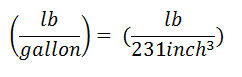# How does the 0.052 constant come from?

In the hydro-static pressure formula, if you use an oil field unit, you must see the hydro-static formula like this

Pressure, psi = 0.052 x Mud Weight (ppg) x TVD (ft)

I got the question asking about how the constant 0.052 is figured out.What is 0.052?

0.052 is a constant used for converting ppg-ft into pound per square inch (lb/in2)

Do you know how it comes from? Let’s get started

First of all, the unit conversions that you need to know are as follows:

1 gallon = 231 cubic inch (inch3)

1 ft = 12 inch

Convert density from ppg (pound per gallon) to lb per cubic inchBasic hydrostatic pressureThe constant can be determined by substituting the unit conversion.The constant is 0.051948 and if we round it up, it will be 0.052.

Share the joyWorking in the oil field and loving to share knowledge.

### 6 Responses to How does the 0.052 constant come from?

1.hamilton says:

Cool 🙂

2.Ahmed says:

Why don’t we consider the gravity acceleration in calculating the mud hydrustatic pressure ?

•DrillingFormulas.Com says:

Ahmed,

This is already accounted for the gravity of acceleration.

3.Candra says:

I have another one
Psi/ppg = 0.433/8,3 =0.051908
Slightly different, is it right?

•DrillingFormulas.Com says:

Once you round up, it is the same figure.

4.Muhammed F. Mousa says:

thanks!

This site uses Akismet to reduce spam. Learn how your comment data is processed.